# Custom Plots¶

You may will sometimes find yourself in a scenario where the standard plots are not a good fit for what you're doing. Fortunately, data visualizations are usually combinations of rectangles, circles, lines, and text; learning how to draw those at specific coordinates is very powerful. In this reading, we'll just focus on text. The challenge will be the coordinates, as there are multiple simultaneous systems in any figure.

As a concrete example, we'll try to reconstruct a very elegant custom plot posted to the /r/dataisbeautiful subreddit:

The original plot looks like this:

In :
from IPython.core.display import Image
Image("reddit.png")

Out: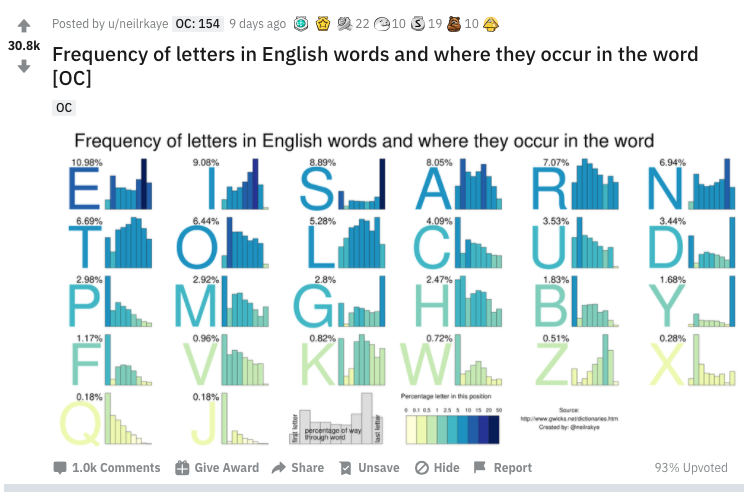The order of the subplots tells you which letters are most common in English words ("E" is most common, representing 10.98% of occurences). The bars in each subplot tell you where those letters occur within the word. Y is almost aways at the end of a word; J is almost always at the beginning.

The version we'll create by the end of the reading will look like this:

In :
Image("reading.png")

Out: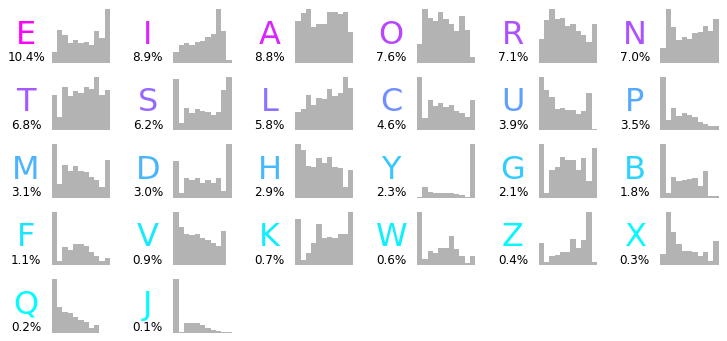## Constructing the Dataset¶

Before we try plotting, we want to build a table where there is one row per letter. Each of ten colunns will represent a location within a word. For example, 0.5 of the A row will tell us how many times the letter A appears in the middle of a word.

We'll can pull the capital letters from the alphabet using Python's builtin string module:

In :
from string import ascii_letters
print(ascii_letters)
caps = list(ascii_letters[26:])
caps[:10]

abcdefghijklmnopqrstuvwxyzABCDEFGHIJKLMNOPQRSTUVWXYZ

Out:
['A', 'B', 'C', 'D', 'E', 'F', 'G', 'H', 'I', 'J']

Let's start with an empty table with the desired rows and columns:

In :
import pandas as pd
df = pd.DataFrame(0, index=caps, columns=[0, 0.1, 0.2, 0.3, 0.4, 0.5, 0.6, 0.7, 0.8, 0.9, 1])

Out:
0.0 0.1 0.2 0.3 0.4 0.5 0.6 0.7 0.8 0.9 1.0
A 0 0 0 0 0 0 0 0 0 0 0
B 0 0 0 0 0 0 0 0 0 0 0
C 0 0 0 0 0 0 0 0 0 0 0
D 0 0 0 0 0 0 0 0 0 0 0
E 0 0 0 0 0 0 0 0 0 0 0

On Linux, the "/usr/share/dict/words" file usually contains a list of English words. We can use the head command to see the first few lines. Putting ! in front of a command in Jupyter means to run this as a shell command (not Python code).

In :
!head /usr/share/dict/words

A
a
aa
aal
aalii
aam
Aani
aardvark
aardwolf
Aaron


If a letter is at index i within a string word, then this will give us the position of that letter in the range 0 (beginning) to 1 (end), rounded to the nearest tenth: round(i / (len(word) - 1), ndigits=1). Let's test that with a simple example.

In :
word = "ABC"
i = 0 # at what percent through the word does A occur?
print(round(i / (len(word) - 1), ndigits=1))
i = 1 # at what percent through the word does B occur?
print(round(i / (len(word) - 1), ndigits=1))
i = 2 # at what percent through the word does C occur?
print(round(i / (len(word) - 1), ndigits=1))

0.0
0.5
1.0


Ok, let's now loop over every letter of every word in the English dictionary, counting it in our big table as we go (df.at[letter, position] += 1).

In :
with open("/usr/share/dict/words") as f:
for word in list(f):
word = word.strip().upper()
if len(word) == 1:
continue
for i in range(len(word)):
letter = word[i]
position = round(i / (len(word) - 1) * 10) / 10
if not letter in caps:
continue
df.at[letter, position] += 1

In :
df.head()

Out:
0.0 0.1 0.2 0.3 0.4 0.5 0.6 0.7 0.8 0.9 1.0
A 17111 20306 21680 14746 15727 15907 20566 20573 19775 20545 12616
B 11068 1080 4285 3411 3812 3854 4102 2401 5502 441 475
C 19905 4555 11380 8937 10067 8836 9381 7208 6651 5192 11326
D 10894 1501 6100 5240 5897 4598 5316 4610 5994 2052 15987
E 8748 27212 23450 16643 19035 16813 17079 14914 26509 20730 44196

Let's sum horizontally along each row, to determine the total number of occurences of each letter. We can divide this by the total count and sort to rank the letters by frequency.

In :
counts = df.sum(axis=1)
percents = counts / counts.sum() * 100
percents = percents.sort_values(ascending=False)

Out:
E    10.425848
I     8.906289
A     8.840809
O     7.562128
R     7.132076
dtype: float64

## Text Annotations: Coordinates and Colors¶

Eventually, we'll create a matrix like the following, using the first 26 subplots for the 26 letters:

In :
%matplotlib inline

In :
import matplotlib.pyplot as plt
fig, axes = plt.subplots(nrows=5, ncols=6, figsize=(12, 6))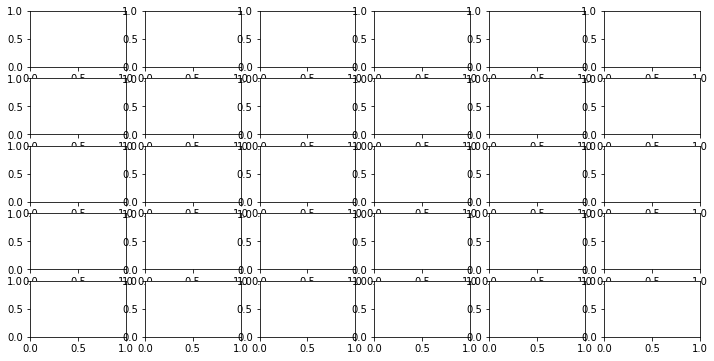For now, let's think about how to plot one letter in one AxesSubplot.

In :
df.head()

Out:
0.0 0.1 0.2 0.3 0.4 0.5 0.6 0.7 0.8 0.9 1.0
A 17111 20306 21680 14746 15727 15907 20566 20573 19775 20545 12616
B 11068 1080 4285 3411 3812 3854 4102 2401 5502 441 475
C 19905 4555 11380 8937 10067 8836 9381 7208 6651 5192 11326
D 10894 1501 6100 5240 5897 4598 5316 4610 5994 2052 15987
E 8748 27212 23450 16643 19035 16813 17079 14914 26509 20730 44196

df.loc[SOME_LETTER] pulls out a row of the big table (corresponding to the given letter) as a Series, which is easy enough to plot in a given ax.

In :
A = df.loc["A"]
A

Out:
0.0    17111
0.1    20306
0.2    21680
0.3    14746
0.4    15727
0.5    15907
0.6    20566
0.7    20573
0.8    19775
0.9    20545
1.0    12616
Name: A, dtype: int64
In :
fig, ax = plt.subplots(figsize=(3,2))
A.plot.bar(ax=ax, color="0.85") # 0.5 is light gray (0 is black, 1 is white)

Out:
<matplotlib.axes._subplots.AxesSubplot at 0x7fe78fdd2518>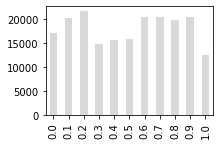If we like, we can add some text using ax.plot(x, y, text).

In :
fig, ax = plt.subplots(figsize=(3,2))
A.plot.bar(ax=ax, color="0.85")
ax.text(3, 16000, "hi", transform=ax.transData, size=20, color="red")

Out:
Text(3, 16000, 'hi')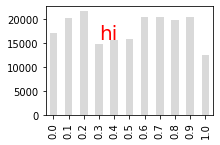The transform=ax.transData (which would have been the default had we exclude it) is telling matplotlib to interpret 3 and 16000 in terms of the scale of the data. 16000 makes sense by looking at the y-axis, but why is it 3 instead of 0.3? Matplotlib is treating the x-axis like a category, even though it looks numeric, as we can see by looking at xlim:

In :
ax.get_xlim()

Out:
(-0.5, 10.5)

ax.transAxes is a different coordinate system. The bottom left of the subplot is x=0, y=0; the top right is x=1, y=1. This is convenient, say, if we want to center the text (note that we're centering the bottom left of the text).

In :
fig, ax = plt.subplots(figsize=(3,2))
A.plot.bar(ax=ax, color="0.85")
ax.text(0.5, 0.5, "hi", transform=ax.transAxes, size=20, color="red")

Out:
Text(0.5, 0.5, 'hi')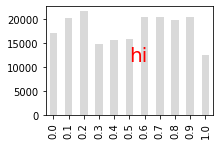We can specify a negative x coord in the ax.transAxes coordinate system to make the text float to the left of the subplot. Notice now that we're specifying the coordinates for the center (rather than the bottom left) by specifying alignment.

In :
fig, ax = plt.subplots(figsize=(3,2))
A.plot.bar(ax=ax, color="0.85")
ax.text(-0.4, 0.5, "A", size=32,
verticalalignment="center", horizontalalignment="center",
transform=ax.transAxes)

Out:
Text(-0.4, 0.5, 'A')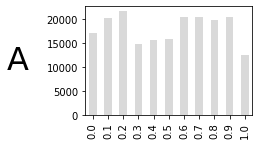Matplotlib has many colormaps that allow us to convert a quantity to point on a color bar: https://matplotlib.org/stable/gallery/color/colormap_reference.html. Colors are represented as tuples.

In :
print(plt.cm.cool(0))
print(plt.cm.cool(0.5))
print(plt.cm.cool(1))

(0.0, 1.0, 1.0, 1.0)
(0.5019607843137255, 0.4980392156862745, 1.0, 1.0)
(0.00392156862745098, 0.996078431372549, 1.0, 1.0)


We can use these colors for our text.

In :
fig, ax = plt.subplots(figsize=(3,2))
A.plot.bar(ax=ax, color="0.85")
ax.text(-0.4, 0.3, "A", size=32, transform=ax.transAxes, color=plt.cm.cool(0))
ax.text(-0.4, 0.5, "B", size=32, transform=ax.transAxes, color=plt.cm.cool(0.5))
ax.text(-0.4, 0.7, "C", size=32, transform=ax.transAxes, color=plt.cm.cool(1.0))

Out:
Text(-0.4, 0.7, 'C')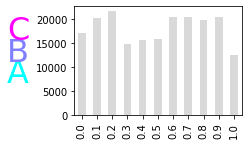We can use these colors to indicate something about the data (like letter frequency).

## Letter Frequencies: Putting it All Together¶

We've seen how we can pull the info for a given letter necessary to create a bar plot showing the frequency of different locations. We've also seen how we can use the ax.text and the ax.transAxes coordinate system to add some text to the left of a subplot. We're going to need to create 26 such subplots, so lets put together all that we've done so far into a plot_letter function. We pass in letter, and the function directly grabs the relevant data from our df earlier in the notebook. We also pass in ax so that the function knows where to plot.

In :
def plot_letter(letter, ax):
percent = percents.at[letter]
color = plt.cm.cool(percent / percents.max())
positions = df.loc[letter]
positions = positions / positions.sum() * 100
positions = positions[:10]
ax.text(-0.4, 0.5, letter, size=32,
verticalalignment="center", horizontalalignment="center",
transform=ax.transAxes, color=color)
ax.text(-0.4, 0.1, str(round(percent, 1)) + "%", size=12,
horizontalalignment="center", verticalalignment="center",
transform=ax.transAxes)
positions.plot.bar(ax=ax, width=1, color="0.7")

In :
fig, axes = plt.subplots(ncols=2, figsize=(6, 2))
plot_letter("H", axes)
plot_letter("I", axes)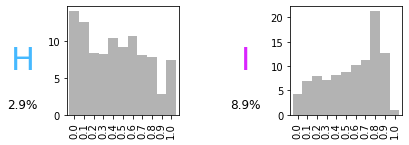We see we can use our plot_letter function to plots "H" and "I" in two subplots. Let's try the whole alphabet. Also, we can now get rid of the axes/borders using ax.axis("off") for a cleaner plot.

In :
fig, axes = plt.subplots(nrows=5, ncols=6, figsize=(12, 6))
axes = list(axes.reshape(-1))

for i in range(len(percents)):
letter = percents.index[i]
plot_letter(letter, axes[i])

for ax in axes:
ax.axis("off")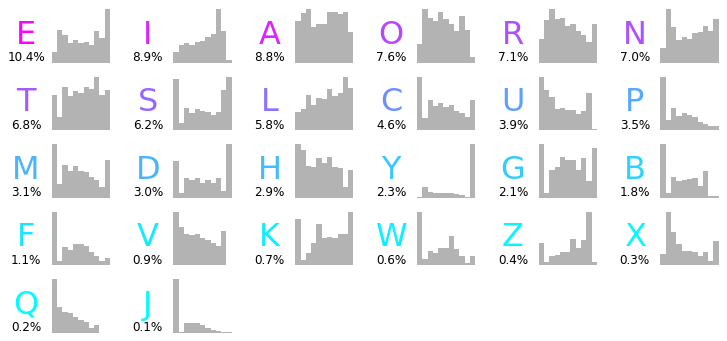Finally, let's save our work.

In :
fig.savefig("reading.png", bbox_inches="tight")